# 5.3 Solve systems of equations by elimination  (Page 3/6)

 Page 3 / 6

Solve the system by elimination. $\left\{\begin{array}{c}3x-4y=-9\hfill \\ 5x+3y=14\hfill \end{array}$

$\left(1,3\right)$

Solve the system by elimination. $\left\{\begin{array}{c}7x+8y=4\hfill \\ 3x-5y=27\hfill \end{array}$

$\left(4,-3\right)$

When the system of equations contains fractions, we will first clear the fractions by multiplying each equation by its LCD.

Solve the system by elimination. $\left\{\begin{array}{c}x+\frac{1}{2}y=6\hfill \\ \frac{3}{2}x+\frac{2}{3}y=\frac{17}{2}\hfill \end{array}$

## Solution

In this example, both equations have fractions. Our first step will be to multiply each equation by its LCD to clear the fractions.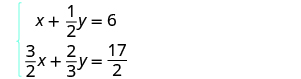To clear the fractions, multiply each equation by its LCD.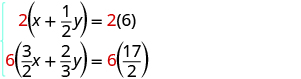Simplify.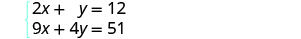Now we are ready to eliminate one of the variables. Notice that both equations are in standard form. We can eliminate y multiplying the top equation by −4.Simplify and add. Substitute x = 3 into one of the original equations.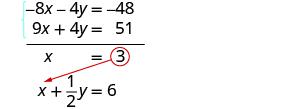Solve for y .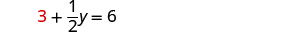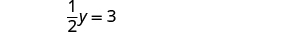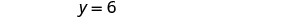Write the solution as an ordered pair. The ordered pair is (3, 6). Check that the ordered pair is a solution to both original equations. $\begin{array}{cccc}\begin{array}{ccc}\hfill x+\frac{1}{2}y& =\hfill & 6\hfill \\ \hfill 3+\frac{1}{2}\left(6\right)& \stackrel{?}{=}\hfill & 6\hfill \\ \hfill 3+6& \stackrel{?}{=}\hfill & 6\hfill \\ \hfill 6& =\hfill & 6\phantom{\rule{0.2em}{0ex}}✓\hfill \\ \\ \\ \\ \\ \end{array}& & & \begin{array}{ccc}\hfill \frac{3}{2}x+\frac{2}{3}y& =\hfill & \frac{17}{2}\hfill \\ \hfill \frac{3}{2}\left(3\right)+\frac{2}{3}\left(6\right)& \stackrel{?}{=}\hfill & \frac{17}{2}\hfill \\ \hfill \frac{9}{2}+4& \stackrel{?}{=}\hfill & \frac{17}{2}\hfill \\ \hfill \frac{9}{2}+\frac{8}{2}& \stackrel{?}{=}\hfill & \frac{17}{2}\hfill \\ \hfill \frac{17}{2}& =\hfill & \frac{17}{2}\phantom{\rule{0.2em}{0ex}}✓\hfill \end{array}\end{array}$ The solution is (3, 6).

Solve the system by elimination. $\left\{\begin{array}{c}\frac{1}{3}x-\frac{1}{2}y=1\hfill \\ \frac{3}{4}x-y=\frac{5}{2}\hfill \end{array}$

$\left(6,2\right)$

Solve the system by elimination. $\left\{\begin{array}{c}x+\frac{3}{5}y=-\frac{1}{5}\hfill \\ -\frac{1}{2}x-\frac{2}{3}y=\frac{5}{6}\hfill \end{array}$

$\left(1,-2\right)$

In the Solving Systems of Equations by Graphing we saw that not all systems of linear equations have a single ordered pair as a solution. When the two equations were really the same line, there were infinitely many solutions. We called that a consistent system. When the two equations described parallel lines, there was no solution. We called that an inconsistent system.

Solve the system by elimination. $\left\{\begin{array}{c}3x+4y=12\hfill \\ y=3-\frac{3}{4}x\hfill \end{array}$

## Solution

$\begin{array}{ccc}& & \phantom{\rule{0.8em}{0ex}}\left\{\begin{array}{ccc}\hfill 3x+4y& =\hfill & 12\hfill \\ \hfill y& =\hfill & 3-\frac{3}{4}x\hfill \end{array}\hfill \\ \\ \\ \text{Write the second equation in standard form.}\hfill & & \phantom{\rule{0.8em}{0ex}}\left\{\begin{array}{ccc}\hfill 3x+4y& =\hfill & 12\hfill \\ \hfill \frac{3}{4}x+y& =\hfill & 3\hfill \end{array}\hfill \\ \\ \\ \begin{array}{c}\text{Clear the fractions by multiplying the}\hfill \\ \text{second equation by 4.}\hfill \end{array}\hfill & & \left\{\begin{array}{ccc}\hfill 3x+4y& =\hfill & 12\hfill \\ \hfill 4\left(\frac{3}{4}x+y\right)& =\hfill & 4\left(3\right)\hfill \end{array}\hfill \\ \\ \\ \text{Simplify.}\hfill & & \phantom{\rule{1em}{0ex}}\left\{\begin{array}{ccc}\hfill 3x+4y& =\hfill & 12\hfill \\ \hfill 3x+4y& =\hfill & 12\hfill \end{array}\hfill \\ \\ \\ \begin{array}{c}\text{To eliminate a variable, we multiply the}\hfill \\ \text{second equation by −1.}\hfill \end{array}\hfill & & \begin{array}{c}\phantom{\rule{0.2em}{0ex}}\underset{\text{________________}}{\left\{\begin{array}{ccc}\hfill 3x+4y& =\hfill & 12\hfill \\ \hfill -3x-4y& =\hfill & -12\hfill \end{array}}\hfill \\ \hfill 0=0\hfill \end{array}\hfill \\ \text{Simplify and add.}\hfill & \end{array}$

This is a true statement. The equations are consistent but dependent. Their graphs would be the same line. The system has infinitely many solutions.

After we cleared the fractions in the second equation, did you notice that the two equations were the same? That means we have coincident lines.

Solve the system by elimination. $\left\{\begin{array}{c}5x-3y=15\hfill \\ y=-5+\frac{5}{3}x\hfill \end{array}$

infinitely many solutions

Solve the system by elimination. $\left\{\begin{array}{c}x+2y=6\hfill \\ y=-\frac{1}{2}x+3\hfill \end{array}$

infinitely many solutions

Solve the system by elimination. $\left\{\begin{array}{c}-6x+15y=10\hfill \\ 2x-5y=-5\hfill \end{array}$

## Solution

$\begin{array}{cc}\text{The equations are in standard form.}\hfill & \phantom{\rule{0.1em}{0ex}}\left\{\begin{array}{ccc}\hfill -6x+15y& =\hfill & 10\hfill \\ \hfill 2x-5y& =\hfill & -5\hfill \end{array}\hfill \\ \\ \\ \begin{array}{c}\text{Multiply the second equation by 3 to}\hfill \\ \text{eliminate a variable.}\hfill \end{array}\hfill & \phantom{\rule{0.1em}{0ex}}\left\{\begin{array}{ccc}\hfill -6x+15y& =\hfill & 10\hfill \\ \hfill 3\left(2x-5y\right)& =\hfill & 3\left(-5\right)\hfill \end{array}\hfill \\ \\ \\ \text{Simplify and add.}\hfill & \begin{array}{c}\underset{\text{__________________}}{\left\{\begin{array}{ccc}\hfill -6x+15y& =\hfill & \phantom{\rule{0.5em}{0ex}}10\hfill \\ \hfill 6x-15y& =\hfill & -15\hfill \end{array}}\\ \hfill 0\ne -5\hfill \end{array}\hfill \end{array}$

This statement is false. The equations are inconsistent and so their graphs would be parallel lines.

The system does not have a solution.

Solve the system by elimination. $\left\{\begin{array}{c}-3x+2y=8\hfill \\ 9x-6y=13\hfill \end{array}$

no solution

Solve the system by elimination. $\left\{\begin{array}{c}7x-3y=-2\hfill \\ -14x+6y=8\hfill \end{array}$

no solution

## Solve applications of systems of equations by elimination

Some applications problems translate directly into equations in standard form, so we will use the elimination method to solve them. As before, we use our Problem Solving Strategy to help us stay focused and organized.

Aziza is solving this equation-2(1+x)=4x+10
No. 3^32 -1 has exactly two divisors greater than 75 and less than 85 what is their product?
x^2+7x-19=0 has Two solutions A and B give your answer to 3 decimal places
3. When Jenna spent 10 minutes on the elliptical trainer and then did circuit training for20 minutes, her fitness app says she burned 278 calories. When she spent 20 minutes onthe elliptical trainer and 30 minutes circuit training she burned 473 calories. How manycalories does she burn for each minute on the elliptical trainer? How many calories doesshe burn for each minute of circuit training?
.473
Angelita
?
Angelita
John left his house in Irvine at 8:35 am to drive to a meeting in Los Angeles, 45 miles away. He arrived at the meeting at 9:50. At 3:30 pm, he left the meeting and drove home. He arrived home at 5:18.
p-2/3=5/6 how do I solve it with explanation pls
P=3/2
Vanarith
1/2p2-2/3p=5p/6
James
Cindy
4.5
Ruth
is y=7/5 a solution of 5y+3=10y-4
yes
James
Cindy
Lucinda has a pocketful of dimes and quarters with a value of $6.20. The number of dimes is 18 more than 3 times the number of quarters. How many dimes and how many quarters does Lucinda have? Rhonda Reply Find an equation for the line that passes through the point P ( 0 , − 4 ) and has a slope 8/9 . Gabriel Reply is that a negative 4 or positive 4? Felix y = mx + b Felix if negative -4, then -4=8/9(0) + b Felix -4=b Felix if positive 4, then 4=b Felix then plug in y=8/9x - 4 or y=8/9x+4 Felix Macario is making 12 pounds of nut mixture with macadamia nuts and almonds. macadamia nuts cost$9 per pound and almonds cost $5.25 per pound. how many pounds of macadamia nuts and how many pounds of almonds should macario use for the mixture to cost$6.50 per pound to make?
Nga and Lauren bought a chest at a flea market for $50. They re-finished it and then added a 350 % mark - up Makaila Reply$1750
Cindy
the sum of two Numbers is 19 and their difference is 15
2, 17
Jose
interesting
saw
4,2
Cindy
Felecia left her home to visit her daughter, driving 45mph. Her husband waited for the dog sitter to arrive and left home 20 minutes, or 13 hour later. He drove 55mph to catch up to Felecia. How long before he reaches her?
hola saben como aser un valor de la expresión
NAILEA
integer greater than 2 and less than 12
2 < x < 12
Felix
I'm guessing you are doing inequalities...
Felix
Actually, translating words into algebraic expressions / equations...
Felix
hi
Darianna
hello
Mister
Eric here
Eric
6
Cindy

#### Get Jobilize Job Search Mobile App in your pocket Now!By RhodesBy Cath YuBy Jonathan LongBy Janet ForresterBy OpenStaxBy Saylor FoundationBy OpenStaxBy OpenStaxBy Jazzycazz JacksonBy John Gabrieli